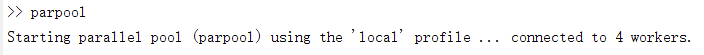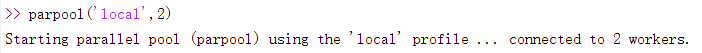# Matlab并行编程

###### 1. 并行方法

parpool  %开启并行池（parallel pool）
%parallel program
delete(gcp('nocreate'))  % 关闭并行池parpool('local',2)PS：Matlab之前的并行方法 matlabpool local 2 已经过时，现在新版的Matlab要用parpool

###### 2. parfor

parfor(parallel for-loop)用于将for循环并行处理，当你的for循环每次迭代计算量很大，并且每次迭代都是独立的话，就可以用parpool来加速，下面是一个例子：

tic
n = 200;
A = 500;
a = zeros(n);
for i = 1:n
a(i) = max(abs(eig(rand(A))));
end
toc

tic
n = 200;
A = 500;
a = zeros(n);
parfor i = 1:n
a(i) = max(abs(eig(rand(A))));
end
toc

###### 3. spmd

spmd(single program multiple data)使多个worker运行同一段代码(single program)，但是不同的worker处理不同的数据(multiple data)，所以spmd适用于处理很大的数据。常见的情形如：

• Programs that take a long time to execute — spmd lets several workers compute solutions simultaneously.

• Programs operating on large data sets — spmd lets the data be distributed to multiple workers.

spmd (3)
R = rand(4,4);
end

spmd (3)
if labindex==1
R = rand(9,9);
else
R = rand(4,4);
end
end

spmd (3)
result = MyFunction(labdata)
end

Composite01-0311万+
11-03
02-191万+
03-251341
11-264893
08-095万+
10-082273
10-231979
05-314万+
04-09178
06-24615
10-242201
12-064634
12-066429
07-254383
02-272942
06-283万+
07-132万+